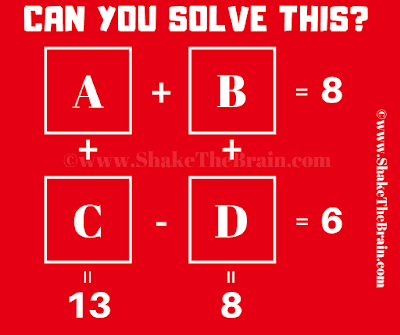Test your Mathematical skills with this Math Puzzle. In this Maths Puzzle, four equations are given and there are four variables A, B, C, and D. Can you solve these equations and find values of A, B, C, and D?Can you find values of A, B, C, and D?

The answer to this Math Puzzle for Teens can be viewed by clicking on the button. Please do give your best try before looking at the answer.

#### 1 comment:

S Ramanujam said...

Good. I want plenty more